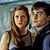# We Bet You Can't Score Over 80% on This Difficult Algebra Quiz

## Algebra, yes can be hard, but it's not impossible. Those who study math could probably beat this quiz without a hitch. Can you?Created by Carly Wallace
On May 13, 2023

How did you do in math in high school? Could you solve algebra equations easily? Algebra can be difficult! Not many people can solve this simple algebra quiz. Scoring 80% is near impossible! Can you solve x for the following equations? See if you can do it without a calculator... (cause.. you know.. that's cheating). 🤪

>> This Mental Math Test Is Almost Impossible to Pass. Let's See How You Do <<

1 / 10

"Solve for x"

3x = 7

2 / 10

"Solve for x"

2x + 1 = 0﻿

3 / 10

"Solve for x"
﻿
x + 5 = 12

4 / 10

"Solve for x"

8x + 5 = 21

5 / 10

"Solve for x"

6x -(-1) = 55

6 / 10

"Solve for x"

x + 9 = 18

7 / 10

"Solve for x"

-3 + 2x = 11

8 / 10

"Solve for x"

4x + 6 = -10

9 / 10

"Solve for x"

2x + 6 = 4x - 2

10 / 10

"Solve for x"

12x + 0 = 144

10
Questions left

### What type of math are you best at?

Calculating results
These are 10 of the World CRAZIEST Ice Cream Flavors
Created by Tal Garner
On Nov 18, 2021